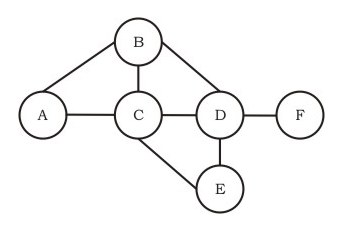# GATE | Gate IT 2005 | Question 91

• Difficulty Level : Hard
• Last Updated : 28 Jun, 2021

Q85 Part_B

Consider a simple graph with unit edge costs. Each node in the graph represents a router. Each node maintains a routing table indicating the next hop router to be used to relay a packet to its destination and the cost of the path to the destination through that router. Initially, the routing table is empty. The routing table is synchronously updated as follows. In each updation interval, three tasks are performed.

1. A node determines whether its neighbours in the graph are accessible. If so, it sets the tentative cost to each accessible neighbour as 1. Otherwise, the cost is set to ∞.
2. From each accessible neighbour, it gets the costs to relay to other nodes via that neighbour (as the next hop).
3. Each node updates its routing table based on the information received in the previous two steps by choosing the minimum cost.For the graph given above, possible routing tables for various nodes after they have stabilized, are shown in the following options. Identify the correct table.
1)Table for node A
 A – – B B 1 C C 1 D B 3 E C 3 F C 4
2)Table for node C
 A A 1 B B 1 C – – D D 1 E E 1 F E 3
3)
Table for node B
 A A 1 B – – C C 1 D D 1 E C 2 F D 2
4)Table for node D
 A B 3 B B 1 C C 1 D – – E E 1 F F 1

Continuing from the earlier problem, suppose at some time t, when the costs have stabilized, node A goes down. The cost from node F to node A at time (t + 100) is

(A) >100 but finite
(B)
(C) 3
(D) >3 and ≤100